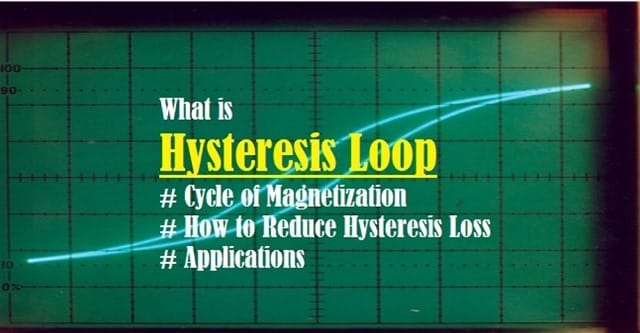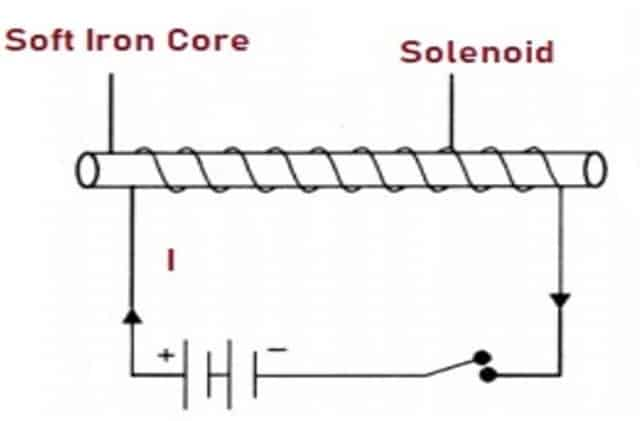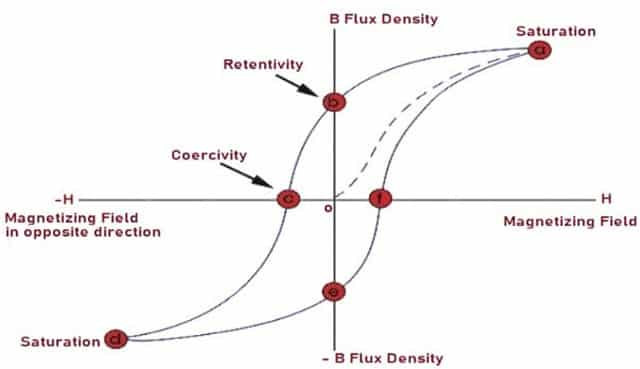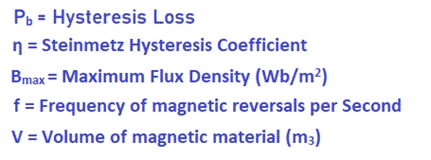Friday, January 27, 2023
HomeHysteresis LoopHysteresis Loop-Definition, BH Curve Magnetization Cycle Hysteresis Loss

# Hysteresis Loop-Definition, BH Curve Magnetization Cycle Hysteresis Loss

Hysteresis Loop also called Hysteresis Curve or BH Curve shows the relationship between the induced magnetic flux density and the magnetizing force. This post will discuss about what is Hysteresis Loop (BH Curve), Definition, Cycle of Magnetization, Hysteresis Loss and how to reduce it.

## What is Hysteresis Loop – Definition of BH Curve

The phenomenon of lagging of intensity of Magnetization (I) or Magnetic Induction (B) behind magnetizing field (H), when a Ferro-magnetic material like iron is subjected to a complete cycle of magnetization is called as Hysteresis.Fig. 1 – Introduction to Hysteresis Loop

When we place an iron rod inside a current carrying conductor, the iron rod attains magnetic property due to the magnetic field in the conductor. However, when we reverse the current, then the iron rod gets demagnetized. This cycle of Magnetization represents Hysteresis Curve or Loop.

The BH Curve exhibits the relation between the induced Magnetic Flux density (B) and the Magnetizing Force (H). Also, The shape of the BH Curve is different for various ferro-magnetic materials as shown in the Fig. 2. This shape of the curve determines the magnetic properties of the material and its ability to retain magnetization.Fig. 2 – BH Curve for (a) Soft Iron (b) Hard Steel

## Relation between Magnetization Cycle and Hysteresis

Let us consider an iron core placed in a solenoid as shown in the Fig. 3. When we increase the current through Solenoid, it also increases the magnetic intensity. We can represent this generated magnetic field as H. As a result, Magnetic flux density B also increases with the increase in H. This is represented by the curve OA of Hysteresis Loop in Fig. 4.

The current is further increased through solenoid. At this point, the value of Magnetizing field increases but there will be no change in Magnetic flux density B. This is known as point of saturation ‘A’ as shown in the Fig. 4.Fig. 3 – Iron Core in Solenoid

### Process of Retentivity

Now, we reduce the value of current in the solenoid till H = 0. From here, De-magnetization of the iron starts. Even when the Magnetizing field is ‘0’ or withdrawn, Magnetic flux density remains and is not equal to ‘0’. This shows that the iron core retains some Magnetization (OB) and we know this residual magnetization as “Retentivity”.

### Process of Coercivity

To remove this residual magnetization, we can reverse the direction of current through Solenoid such that H acts in the opposite direction represented as ‘OC’. This reverse magnetizing field (OC) reduces Magnetizing field to ‘0’ so that the material does not have any magnetization. We known this property as “Coercivity“.

### The BH Curve

From this point, we increase the value of current further which in turn increases reverse magnetizing field. Accordingly, value of B also increases in the opposite direction till it attains Saturation point ‘D’. Now if the decrease reverse magnetizing field gradually, flux density will also decrease as depicted by the curve ‘DE’. There is residual magnetization ‘OE’ at H = 0 and B ≠ 0. To remove this residual magnetization, we will apply forward magnetizing field as represented by the curve ‘EF’.

We call this complete loop “abcdefa” as Cycle of Magnetization. Also, the Cycle of Magnetization shows that Magnetic Induction lags the magnetizing field producing it. We know this phenomenon as Hysteresis and the curve formed is known as BH Curve or Hysteresis Loop.Fig. 4 – BH Curve or Hysteresis Loop

## Hysteresis Loss Definition

We can define Hysteresis Loss as the energy required to go through one complete cycle of BH Curve. The Magnetization Cycle spends energy which appears in the form of heat. This heat loss is termed as Hyst. loss. The area of the BH Curve represents the loss of energy per unit volume of the substance.

The equation for Hyst. Loss is :Where,## How to Reduce Hysteresis Loss

We can reduce the losses effectively by using materials with high permeability and low coercive force values such as:

• Soft Iron
• Iron-Nickel Alloys
• Amorphous Magnetic Alloys

## Applications of Hysteresis Loop

The applications include:

• Soft Iron has narrow BH Curve and hence are widely used in Transformer Cores.
• The area of Hysteresis Loop for Steel is large and that’s why they have their utilization in making Permanent Magnets.
• We select materials for magnetic recording, memory devices, AC electrical equipment etc. on the basis of the area of the BH Curve of different ferro-magnetic materials.

## Advantages of Hysteresis Loop (BH Curve)

• Hysteresis Loop helps in estimating residual magnetism.
• Small area of the loop indicates less Hyst. loss.

• If the area of the loop is more, then Hysteresis Loss is also large.
• This loss increases with increase in frequency which results in reduced efficiency of the output power.
```Also Read:
Hall Effect Principle - History, Theory Explanation, Mathematical Expressions
Electric Vehicle - Range, Charging Stations for Electric Vehicles```Laxmi Ashrit
Laxmi is a B.E (Electronics & Communication) and has work experience in RelQ Software as Test Engineer and HP as Technical support executive. She is an author, editor and partner at Electricalfundablog.
RELATED ARTICLES